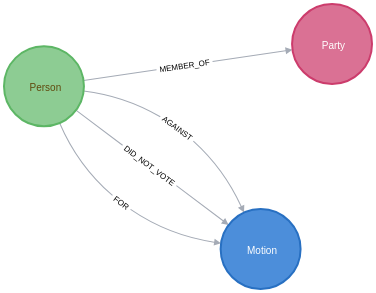# Graphing Brexit: Plotting how the parties voted

Over the last week I’ve revisited the Brexit Graph that I created in March 2019, this time looking at how the parties voted on average on each of the indicative votes.

To recap, we have a graph that has the following schema:Since the initial post I’ve slightly changed how the `MEMBER_OF` relationship works. As several MPs have switched MPs in the intervening months, we’re now storing a `start` property to indicate when they started representing a party and an `end` property to indicate when they stopped reprsenting a party. This change to the model means that we need to do some filtering to work out which party they were representing when a vote took place.

We want to work out how the parties voted on average across each of the different Brexit indicative votes. We want to come up with a score for each party for each vote, which we’ll do by aggregating scores by party using the following scoring system for each MP’s position on a particular motion:

• Voted For = 1.0

• Did Not Vote = 0.5

• Voted Against = 0

Let’s run through a couple of examples:

• If everybody in a party voted `For` a motion, that party would have a score of 1.0 for that motion.

• If everybody in a party voted `Against` a motion, that party would have a score of 0.0 for that motion.

• If a party has 5 MPs, with 3 voting `For` and 2 `Did Not Vote`, they’d have a score of `(3*1.0 + 2*0.5)/5 = 4/5 = 0.8`

We can compute the average vote by a party for a motion by writing the following query:

``````MATCH (m:Motion)<-[vote]-(person:Person)
MATCH (person)-[memberOf:MEMBER_OF]->(party)
WHERE memberOf.start <= m.date AND (not(exists(memberOf.end)) OR m.date <= memberOf.end)
WITH m, party, CASE WHEN type(vote) = "FOR" THEN 1
WHEN type(vote) = "DID_NOT_VOTE" THEN 0.5
ELSE 0 END AS score
RETURN m.division, m.name, party.name, avg(score) AS score, count(*) AS count
ORDER BY m.name, party.name
LIMIT 10``````
Table 1. Results
m.division m.name party.name score count

"388"

"George Eustice’s motion H (EFTA and EEA)"

"Conservative"

0.2731629392971245

313

"388"

"George Eustice’s motion H (EFTA and EEA)"

"DUP"

0.5

10

"388"

"George Eustice’s motion H (EFTA and EEA)"

"Green"

0.0

1

"388"

"George Eustice’s motion H (EFTA and EEA)"

"Independent"

0.15

20

"388"

"George Eustice’s motion H (EFTA and EEA)"

"Labour"

0.25352112676056326

213

"388"

"George Eustice’s motion H (EFTA and EEA)"

"Labour/Co-operative"

0.265625

32

"388"

"George Eustice’s motion H (EFTA and EEA)"

"Liberal Democrat"

0.4090909090909091

11

"388"

"George Eustice’s motion H (EFTA and EEA)"

"Plaid Cymru"

0.5

4

"388"

"George Eustice’s motion H (EFTA and EEA)"

"Scottish National Party"

0.014285714285714292

35

"388"

"George Eustice’s motion H (EFTA and EEA)"

"Sinn Féin"

0.5

7

None of the parties much liked this motion. The best average score it received was by the DUP, Plaid Cymru, and Sinn Fein, whose members on average didn’t vote. For the other parties the average score indicates that at least some of their members were against it.

We’ll store this information in our graph, by creating a relationship between the party and the motion, storing the average rating as a property on the relationship:

``````MATCH (m:Motion)<-[vote]-(person:Person)
MATCH (person)-[memberOf:MEMBER_OF]->(party)
WHERE memberOf.start <= m.date AND (not(exists(memberOf.end)) OR m.date <= memberOf.end)
WITH m, party, CASE WHEN type(vote) = "FOR" THEN 1
WHEN type(vote) = "DID_NOT_VOTE" THEN 0.5
ELSE 0 END AS score
WITH m, party, avg(score) AS score
MERGE (party)-[averageVote:AVERAGE_VOTE]->(m)
SET averageVote.score = score;``````

The image below shows the relationships that have been created:Now that we’ve stored the average votes, we can write a bit of Python code to create bar charts showing the average votes across all the motions. The following code does this:

``````import matplotlib.pyplot as plt
import numpy as np
from neo4j import GraphDatabase

driver = GraphDatabase.driver("bolt://localhost")

fig = plt.figure()
fig.set_size_inches(16.5, 14.5)

with driver.session() as session:
for index, division in enumerate(["386", "387", "388", "389", "390", "391", "392", "393"]):
rows = session.run("""
MATCH (m:Motion {division: \$division})
RETURN m.name AS motion
""", {"division": division})
motion = rows.peek()["motion"]

rows = session.run("""
MATCH (p:Party)-[vote:AVERAGE_VOTE]->(m:Motion {division: \$division})
RETURN p.name AS party, vote.score AS score, m.name AS motion
ORDER BY party
""", {"division": division})

result = [{"party": row["party"], "score": row["score"]} for row in rows ]

plt.rcdefaults()

parties = [item["party"] for item in result]
y_pos = np.arange(len(parties))
scores = [item["score"] for item in result]

ax.barh(y_pos, scores, align='center')
ax.set_yticks(y_pos)
ax.set_yticklabels(parties)
ax.invert_yaxis()

ax.set_title(motion)

ax.set_xlim([0,1])
plt.xticks([0, 0.5, 1], ["Against", "Did Not Vote", "For"])

plt.tight_layout()